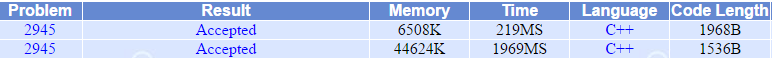# poj_2945 Find the Clones （Trie树 内存分配）

【题目描述】

Doubleville, a small town in Texas, was attacked by the aliens. They have abducted some of the residents and taken them to the a spaceship orbiting around earth. After some (quite unpleasant) human experiments, the aliens cloned the victims, and released multiple copies of them back in Doubleville. So now it might happen that there are 6 identical person named Hugh F. Bumblebee: the original person and its 5 copies. The Federal Bureau of Unauthorized Cloning (FBUC) charged you with the task of determining how many copies were made from each person. To help you in your task, FBUC have collected a DNA sample from each person. All copies of the same person have the same DNA sequence, and different people have different sequences (we know that there are no identical twins in the town, this is not an issue).

【题目要求】

The input contains several blocks of test cases. Each case begins with a line containing two integers: the number 1 ≤ n ≤ 20000 people, and the length 1 ≤ m ≤ 20 of the DNA sequences. The next n lines contain the DNA sequences: each line contains a sequence of m characters, where each character is either A', C', G' or T'.
The input is terminated by a block with n = m = 0 .

For each test case, you have to output n lines, each line containing a single integer. The first line contains the number of different people that were not copied. The second line contains the number of people that were copied only once (i.e., there are two identical copies for each such person.) The third line contains the number of people that are present in three identical copies, and so on: the i -th line contains the number of persons that are present in i identical copies. For example, if there are 11 samples, one of them is from John Smith, and all the others are from copies of Joe Foobar, then you have to print 1' in the first andthe tenth lines, and 0' in all the other lines.

【样例输入】

9 6
AAAAAA
ACACAC
GTTTTG
ACACAC
GTTTTG
ACACAC
ACACAC
TCCCCC
TCCCCC
0 0

【样例输出】

1
2
0
1
0
0
0
0
0

【我的解法】

#include <stdio.h>

typedef struct
{
char c;
int num;
long int fChild,rCousin;
} node;

void newNode(node tree[], long int p, char ch)
{
tree[p].c=ch;
tree[p].num=0;
tree[p].fChild=-1;
tree[p].rCousin=-1;
}

void print(node tree[], long int p, int a[])
{
a[tree[p].num]++;
if (tree[p].fChild>0) print(tree,tree[p].fChild,a);
if (tree[p].rCousin>0) print(tree,tree[p].rCousin,a);
}

int main()
{
node tree;
int m,n;
scanf("%d%d",&n,&m);
while (n>0)
{
long int total=0,i,j,k;
char s;
newNode(tree,0,'0');
for (i=0;i<n;i++)
{
scanf("%s",s);
long int x=0,y;
for (j=0;j<m;j++)
{
y=tree[x].fChild;
if (y==-1)
{
for (k=j;k<m;k++)
{
total++;
newNode(tree,total,s[k]);
tree[x].fChild=total;
x=total;
}
break;
}
while (y!=-1)
{
if (tree[y].c==s[j]){x=y;break;}
x=y;
y=tree[y].rCousin;
}
if (y==-1)
{
total++;
newNode(tree,total,s[j]);
tree[x].rCousin=total;
x=total;
for (k=j+1;k<m;k++)
{
total++;
newNode(tree,total,s[k]);
tree[x].fChild=total;
x=total;
}
break;
}
}
tree[x].num++;
}
int a={0};
print(tree,0,a);
for (i=1;i<=n;i++) printf("%d\n",a[i]);
scanf("%d%d",&n,&m);
}
return 0;
}

#include <stdio.h>
#include <stdlib.h>

typedef struct node
{
char c;
int num;
struct node* fChild;
struct node* rCousin;
}* triTree;

triTree newTree(char ch)
{
triTree p=(triTree) malloc(sizeof(struct node));
p->c=ch;
p->num=0;
p->fChild=NULL;
p->rCousin=NULL;
return p;
}

void print(triTree t, int a[])
{
a[t->num]++;
if (t->fChild!=NULL) print(t->fChild,a);
if (t->rCousin!=NULL) print(t->rCousin,a);
}

int main()
{
int m,n,i,j,k;
char s;
scanf("%d%d",&n,&m);
while (n>0)
{
triTree t=newTree('0');
for (i=0;i<n;i++)
{
scanf("%s",s);
triTree x=t,y;
for (j=0;j<m;j++)
{
y=x->fChild;
if (y==NULL)
{
for (k=j;k<m;k++){x->fChild=newTree(s[k]); x=x->fChild;}
break;
}
while (y!=NULL)
{
if (y->c==s[j]){x=y;break;}
x=y;
y=y->rCousin;
}
if (y==NULL)
{
x->rCousin=newTree(s[j]);
x=x->rCousin;
for (k=j+1;k<m;k++){x->fChild=newTree(s[k]); x=x->fChild;}
break;
}
}
x->num++;
}
int a={0};
print(t,a);
for (i=1;i<=n;i++) printf("%d\n",a[i]);
scanf("%d%d",&n,&m);
}
return 0;
}05-315302-15713
07-30178
12-26850
12-05562
08-14806
10-28456
07-2451
10-27744
07-2821
07-05573
10-31851
05-301009
06-302657
08-1020
07-26370
02-28518
02-26923
02-231136